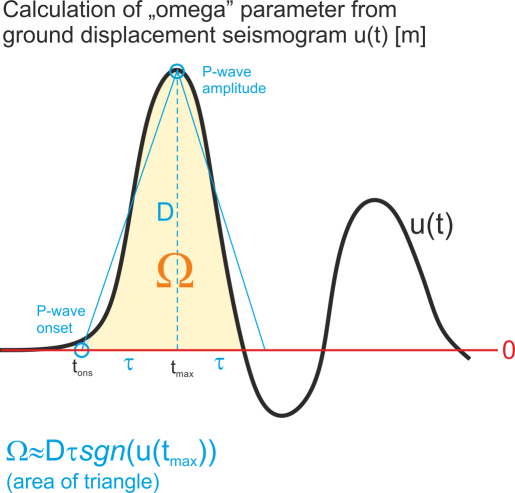# What is the “omega” parameter in the focimt input file and how to calculate it?“omega” parameter which is a part of ASCII input file is basically the area below the first P-wave ground displacement pulse (in time domain) or equivalently the spectral level taken from the amplitude spectrum of ground displacement pulse. The unit of “omega” parameter is [m * s] (meter times second), and it comes from the fact that we multiply the amplitude of first P-wave pulse using ground displacement seismogram in [m] by the half of duration of the first P-wave pulse in [s]. Regardless of whether time or frequency domain was used to extract the “omega” value, the parameter should contain the sign information. The sign of “omega” is in accordance with the seismological convention, i.e. it is positive for the positive first P-wave ground displacement amplitude (i.e. ground motion AWAY from the seismic source) and negative otherwise (ground motion TOWARDS the seismic source).

In case of simple impulsive source time functions it is typically not necessary to calculate the integral of ground displacement first P-wave pulse. Instead, the amplitude D (cf. picture) including sign information of first P-wave pulse may be taken from ground displacement seismogram (the seismogram should be calibrated to [m]) and multiplied by the the rise time (i.e. time interval between the P-wave onset and P-wave amplitude, t_max-t_ons in seconds [s]). In this case, the integral of the pulse is simply replaced by the area of the triangle.